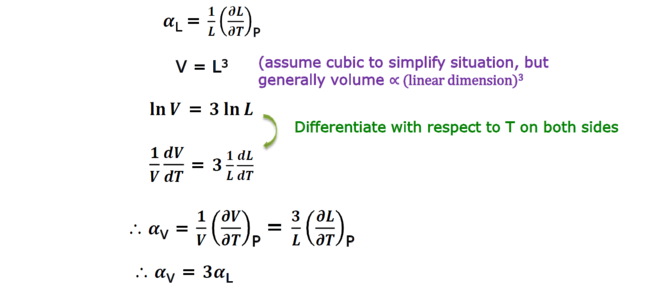6.3

# Thermal expansion coefficient: derivation of relationship between linear expansion and volume expansion

${ \alpha }_{ L }$ VS ${ \alpha }_{ V }$

In calculating entropy change for a solid under isothermal pressure change, we used thermal volume expansion coefficient (${ \alpha }_{ V }$). However, in most cases, not volume but linear expansion coefficients (${ \alpha }_{ L }$) are available. In the video, we used the relationship

${ \alpha }_{ V }$=3${ \alpha }_{ L }$

Here, let’s derive this relationship.